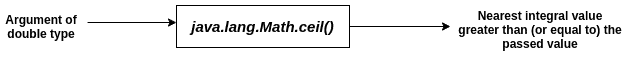# Java ceil() Function: The Complete Guide

Java.lang.Math.ceil(double a) function returns the smallest (closest to negative infinity) double value that is greater than or equal to the argument and is equal to a mathematical integer.

## Java Math ceil()

Java.lang.Math.ceil() is a built-in method used to calculate the nearest integer greater than (or equal to if the passed value is itself an integer) the passed value. Ceil values are often required in various algorithm-building scenarios dealing with mathematical operations and can be directly seen in various mathematical formulae.

1. If the argument value is already equal to a mathematical integer, then the result is the same as the argument.
2. If the argument is NaN or an infinity or positive zero or negative zero, then the result is the same as the argument.
3. If the argument value is less than zero but greater than -1.0, the result is negative zero.

### Syntax

```public static double ceil(double x)
```

### Parameter(s)

The variable of a double type whose ceil value is to be calculated.

### Return Value

For integral values, returns the passed value.

For non-integral values, returns the nearest integer greater than the passed value.

See the following figure.#### Note

1. If the passed value is NaN, infinite, positive zero, or negative zero, then the method returns the same value that is passed.
2. For negative values greater than -1.0, this method returns negative zero.
3. Math.ceil(x) = – Math.floor(-x).

Consider the following examples.

#### Example1.java: The following example demonstrates the use of this method.

```import java.util.*;

public class Example1 {
public static void main(String[] args) {
double a = 1.3;
double b = 2.0;

System.out.println(Math.ceil(a));
System.out.println(Math.ceil(b));
}
}
```

#### Output

```->javac Example1.java
->java Example1
2.0
2.0
```

#### Example2.java: The following example demonstrates the situation when a negative value is passed.

```import java.util.*;

public class Example2 {
public static void main(String[] args) {
double a = -1.3;
System.out.println(Math.ceil(a));

}
}
```

#### Output

```->javac Example2.java
->java Example2
-1.0
```

#### Example3.java: The following example demonstrates the situation of passing NaN, infinite, positive zero, or negative zero values.

```import java.util.*;

public class Example3 {
public static void main(String[] args) {
double a = Double.NaN;
double b = Double.POSITIVE_INFINITY;
double c = Double.NEGATIVE_INFINITY;
double d = 0.0;
double e = -0.0;

System.out.println(Math.ceil(a));
System.out.println(Math.ceil(b));
System.out.println(Math.ceil(c));
System.out.println(Math.ceil(d));
System.out.println(Math.ceil(e));

}
}
```

#### Output

```->javac Example3.java
->java Example3
NaN
Infinity
-Infinity
0.0
-0.0```

#### Example4.java: The following example demonstrates the situation of passing a negative value greater than -1.0

```import java.util.*;

public class Example4 {
public static void main(String[] args) {
double a = -0.3;
System.out.println(Math.ceil(a));
}
}
```

#### Output

```->javac Example4.java
->java Example4
-0.0
```

#### Example5.java: The following example demonstrates the equality of Math.ceil(x) and negative of Math.floor(-x).

```import java.util.*;

public class Example5 {
public static void main(String[] args) {
double a = 2.4;
System.out.println(Math.ceil(a));
System.out.println(-Math.floor(-a));

}
}
```

#### Output

```Output:
->javac Example5.java
->java Example5
3.0
3.0
```

#### Example6.java: The following example demonstrates a scenario where the ceil method can be used. Consider the following problem.

One memory unit can hold at most 8 bits of data. Given the number of bits, calculate the minimum number of memory units needed to store those bits.

```import java.util.*;

public class Example6 {
public static void main(String[] args) {
Scanner sc = new Scanner(System.in);
int bits = sc.nextInt();

System.out.println(Math.ceil((double) bits / 8));
}
}
```

#### Output

```Test Case 1:
Input : 8
Output: 1.0

Test Case 2:
Input : 17
Output: 3.0

```